Courses

XAT Quantitative Ability And DI MCQ Quiz - 1

29 Questions MCQ Test MBA Exams Mock Test Series and Past Year Question Papers | XAT Quantitative Ability And DI MCQ Quiz - 1

Description
Attempt XAT Quantitative Ability And DI MCQ Quiz - 1 | 29 questions in 60 minutes | Mock test for CAT preparation | Free important questions MCQ to study MBA Exams Mock Test Series and Past Year Question Papers for CAT Exam | Download free PDF with solutions
QUESTION: 1

What is the minimum value of (48 cos x - 20 sin x)?

Solution:

Let 48 = r * cos y and 20 = r sin y. 48 cos x - 20 sin x = r(cos y * cos x) - r{sin y * sin x) = r{cos (x + y))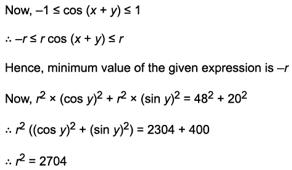r= 52
Hence, minimum value of the given expression is -52.
Hence, option 3.

QUESTION: 2

Group Question  Answer the following question based on the information given below. The following pie-chart shows the sales and revenue collection for 5 different products produced in an electronic company. The cost incurred in producing a T.V., Fridge, Computer, D.V.D. and Micro-wave oven is Rs. 800, Rs. 950, Rs. 1350, Rs. 650 and Rs. 1300 per unit respectively. Profit = Collection - Cost incurred Loss = Cost incurred - Collection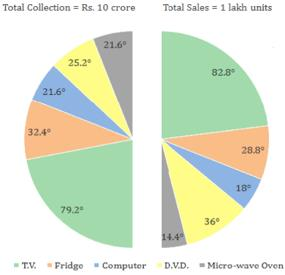Q. What is the profit earned on one unit of Fridge?

Solution: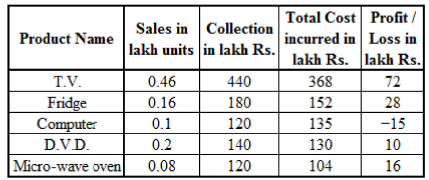Here, the total collection per product and the total sales per product, each occupy exactly one-half of the pie-chart; and the total collection/sales for all 5 products is given at the top of the corresponding half of the pie-chart.  When calculating the percentage of collection/sales for each product out of the total collection/sales for all products, the values given in the pie-chart should be divided by 180°, not 360°.
Consider the following example:

For T.V.
Total sales = 82.8° = (82.8/180) x 100 = 46%. Total sales = 46% of 1 lakh units = 0.46 lakh units Total collection = 79.2° = (79.2/180) x 100 = 44%. Total collection = 44% of 10 crores = Rs. 440 lakhs Total cost incurred in production = 0.46 x 800 = Rs. 368 lakhs.
Total profit = 440 - 368 = Rs. 72 lakhs Thus, the profit per unit can be calculated for each product as shown in the table above.
Profit earned on Fridge per unit = 28/0.16 = Rs. 175

QUESTION: 3

The following pie-chart shows the sales and revenue collection for 5 different products produced in an electronic company. The cost incurred in producing a T.V., Fridge, Computer, D.V.D. and Micro-wave oven is Rs. 800, Rs. 950, Rs. 1350, Rs. 650 and Rs. 1300 per unit respectively. Profit = Collection - Cost incurred Loss = Cost incurred - CollectionQ. What is the difference in the profit earned on Fridge and D.V.D. as a percentage of the profit earned on D.V.D.?

Solution:

Profit earned on Fridge and D.V.D is Rs. 28 lakhs and Rs. 10 lakhs respectively.
Required percentage = [(28 - 10)/10] * 100 = 180% Hence, option 1.

QUESTION: 4

The following pie-chart shows the sales and revenue collection for 5 different products produced in an electronic company. The cost incurred in producing a T.V., Fridge, Computer, D.V.D. and Micro-wave oven is Rs. 800, Rs. 950, Rs. 1350, Rs. 650 and Rs. 1300 per unit respectively.
Profit = Collection - Cost incurred Loss = Cost incurred - CollectionQ. What is the percentage profit earned by the company?

Solution:

Total cost incurred = 368 + 152+135 + 130 + 104 = Rs. 889 lakhs Total collection = Rs. 1000 lakhs Profit percentage = [(1000 - 889)/889] * 100. = 12.48% ⇒ 12.5%
Hence, option 1.

QUESTION: 5

The following pie-chart shows the sales and revenue collection for 5 different products produced in an electronic company. The cost incurred in producing a T.V., Fridge, Computer, D.V.D. and Micro-wave oven is Rs. 800, Rs. 950, Rs. 1350, Rs. 650 and Rs. 1300 per unit respectively.
Profit = Collection - Cost incurred Loss = Cost incurred - CollectionQ. If the income tax paid is [| profit/collection | * 100] %, then for which product will the company pay lowest income tax?

Solution:

Note that only the magnitude of profit is to be considered to calculate the income tax.
Income tax for T.V. = [|72/440| x 100] = 16.36% Similarly, the income tax can be calculated for each product.
For Fridge = 15.55% For Computer = 12.5% For D.V.D. = 7.14% For Micro-wave oven = 13.3% Thus, the company pays the lowest income tax for D.V.Ds. Hence, option 4.

QUESTION: 6

The following pie-chart shows the sales and revenue collection for 5 different products produced in an electronic company. The cost incurred in producing a T.V., Fridge, Computer, D.V.D. and Micro-wave oven is Rs. 800, Rs. 950, Rs. 1350, Rs. 650 and Rs. 1300 per unit respectively.
Profit = Collection - Cost incurred Loss = Cost incurred - CollectionQ. What is the percentage share of Micro-wave ovens in the total profit earned by the company?

Solution:

Consider the table obtained in the solution to the first question.
Total profit earned by the company = 72 + 2 8 - 1 5 + 10 + 16 = Rs. 111 lakhs.
Profit earned on Microwave ovens = Rs. 16 lakhs.
Therefore, share of Micro-wave ovens in the total profit = (16/111) x 100 = 14.4% Hence, option 4.

QUESTION: 7

Group Question

Answer the following question based on the information given below.

A series of positive integers has the following terms: First term: 1; Second term: (2 + 3 + 4); Third term: (5 + 6 + 7 + 8 + 9); and so on.

Q. What is the last number in the nth term of the series?

Solution:

The first term of the series has 1 term, the second term has 3 terms, the third term has 5 terms and so on.
Therefore, the nth term will be having (2n - 1) terms and the first term of the nth term will be 1 more than the last term of the (n - 1)th term of the series.
The series is moving progressively taking every natural number starting with 1, so if we calculate how many terms have been used in all till the (n - 1)th term, we can find out the first term of the nth term which will be 1 more than the sum of all the terms used till the (n - 1)th term.

Number of terms till the (n - 1 )th term = 1 + 3 + 5 + ... + (2n-3)
= (n-1)2
The first term of the nth term = (n - 1 )2 + 1 And number of terms in the nth term = 2n-1 .The last term of the nth term = (n - 1 )2 + 1 + [(2n - 1) - 1 ] = (n - 1 )2 + ( 2n - 1 ) = n2

Hence, option 1.

QUESTION: 8

A series of positive integers has the following terms: First term: 1; Second term: (2 + 3 + 4); Third term: (5 + 6 + 7 + 8 + 9); and so on.

Q. What is the sum of the numbers that form the nth term?

Solution:

First term of the nth term = (n - 1)2 + 1 = n2 + 2 - 2n, And number of terms in the nth term = 2n-1 The numbers that form the /7th term will be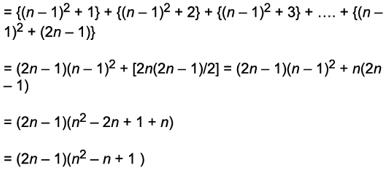QUESTION: 9

What is the total number of natural numbers N such that out of all the factors of N (except 1 and N) the largest factor is 21 times the smallest one?

Solution:

Let S be the smallest factor of N except 1 and L be the largest factor of N except N.
For any natural number N, Smallest factor * largest factor = 2nd smallest factor * 2nd largest factor = 3rd smallest factor x 3rd largest factor = ... = N Thus from the data given in the question and from the above rule, we get, S x L = N, L = 21S. Therefore  S x 21S = N. Therefore 21 S2 = N
Since we want the largest factor (after N) to be 21 times the smallest factor (after 1), we will have to ensure that S takes values less than or equal to the smallest prime factor of 21 i.e. less than or equal to 3.

S = 2, 3
Two such numbers N are possible. And the two values of N are 21 x 22 = 84 and 21 x 32 = 189 Hence, option 2.

QUESTION: 10

A certain biased coin when tossed has a probability p of showing head. If the probability that there will be exactly one head in 5 tosses is same as the probability that there will be exactly two heads in 5 tosses, then what is the probability that there will be exactly 3 heads in 5 tosses?

Solution:

The probability that the coin will show tail = 1 - p. Let us first find the probability of getting exactly one head in 5 tosses.
Let only the first toss show head.
Probability o f this event = p x (1 - p)4 But second, third, fourth or fifth tosses can also show heads.
Hence, probability that there is exactly one head in 5 tosses = 5 x p x (1 - p)4

Similarly, let’s find the probability of getting exactly two heads in 5 tosses.
Let only the first and second tosses show head.
Probability = p2 * (1 - p)3 Now, there are 5C2 different ways in which we get exactly 2 heads in 5 tosses.

Hence, probability that there are exactly 2 heads in 5 tosses = 5C2 x p2 x (1 - p)3 Hence, 5 x p x (1 - p)4 = 5C2 x p2 x (1 -p) 3
1 - p = 2p.

p = 1/3
Now, let’s find the probability that there are exactly 3 heads in 5 tosses.
Let only first three tosses show head.
Hence, probability = p3 * (1 - p)2 But there are 5C3 different ways in which we can get exactly 3 head in 5 tosses.
Hence, probability of getting exactly 3 heads in 5 tosses = 5C3 x p3 x (1 - p)2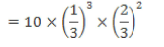= 40/243

Hence, option 3.

QUESTION: 11

Let a, b, c, d be positive real numbers and a + b + c + d=1.  Then (1 - a)(1 - b)(1 - c)(1 - d) is always less than which of the following?

Solution:

a + b + c + d = 1 =>b + c + d = 1 - a.  b + c + d is positive as b, c, and d are positive. (1 - a) is positive.
Similarly, (1 - b), (1 - c) and (1 - d) are positive as a + b + c + d = 1 and a, b, c, d are all positive.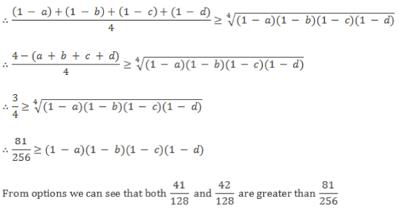So (1 - a)(1 - b)(1 - c)(1 - d) will be always less than both options 2 and 3.

QUESTION: 12

A picks a random integer between 1 and 1499, triples it and gives the result to B. Each time B gets a number from A, adds 100 to the number, and gives the result back to A, who triples the number again. The first person, whose result is more than 2000, loses the game. Let ‘x’ be the smallest initial number that results in a win for A. The sum of the digits of ‘x' is:

Solution:

Let A picks an integer x such that,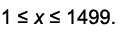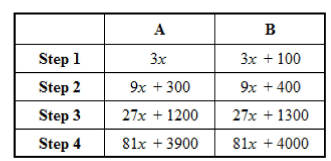A has to win and in step 4, since the result for A goes way above 2000, the game should end in step 3. i.e.,27x + 1300 >2000 i.e., 27x > 700 Least possible value of x is 26.
Also, for x = 26, 27x + 1200 is less than 2000.
Thus, x = 26 and the sum of its digits = 8 Hence, option 3.

QUESTION: 13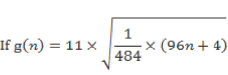where n is a positive integer. Then for how many values of ‘n' is g(n) prime?

Solution: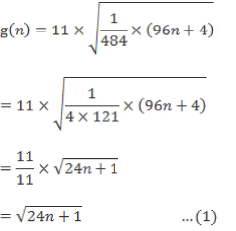We know that every prime number p > 3 can be expressed as 6k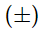1, where 'k' is a natural number.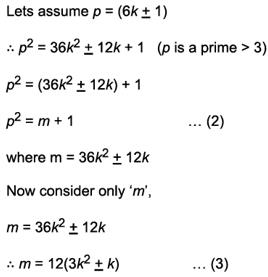3k2k is always even irrespective of whether ‘k' is even or odd. Thus we conclude that ‘m’ will always be even. From this we can further conclude that ‘m’ can be expressed as 24n and thus from the above conclusion and from (2) and (3), we can say that every p2 (where prime number p > 3) can be written as (24n + 1) for some natural number n.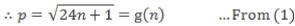∴ g(n) is prime for infinite values of n.

QUESTION: 14

If, m = 2 x 4 x 6 x 8 x ... x 98 x 100. What is the total number of  0’s at the end of m?

Solution:

The number of 0's at the end of m depends on the number of occurrences of pair of 2 and 5 ( 2 x 5 = 10).
Now, m = 250 x (1 x 2 x ... x 49 x 50) m = 250 x 50! .

We can see that we have enough 2’s already and the constraint is on the occurrences of 5.
We calculate the number of occurrences of 5 in 50! as follows: [50/5] + [50/25] + [50/125] = 10 + 2 + 0=12 ...(Here, [x] represents greatest integer less than or equal to x). therefore 12 occurrences of 5 in 50!
There are 12 zeroes at the end of m.
Hence, option 3.

QUESTION: 15

Towns A and B are 200 km apart; A is in the South and B in the North. There is only one railway track between them; on a certain day, trains start simultaneously from both A and B with different speeds towards each other. The train starting from A has a speed of 40 km/hr. A fly is initially 80 km north of A. As soon as the trains start, it starts flying towards the train initially at B; on reaching it, it immediately turns around and starts flying towards the other train, and turns around again on reaching it. It continues this behaviour till the trains meet and it is squashed. At the time that the trains meet, the fly has flown equal distances North and South. What is the speed (in km/hr) of the second train?

Solution:

The fact that the fly has flown equal distances North and South shows that its final position is the same as its initial position; the point where the trains meet is 80 km from A.
The train starting from A has a speed of 40 km/hr, so it takes 2 hours to reach this point.
The other train must also take 2 hours to reach this same point, which is (200 - 80 =) 120 km from B, so the speed of the second train is 120/2 = 60 km/hr.
Hence, option 4.

QUESTION: 16

Three packs of 52 playing cards are shuffled together. In how many ways can 26 cards from this stack be selected such that there is no duplicate card (in these 26 cards)? [Note: Duplicate card means a card of the same suit and same denomination.]

Solution:

There are 52 different type of cards i.e. 52 combinations of suit and denominations. Since no duplicate card is allowed, let us first choose 26 distinct type of cards from these 52 type of cards.
This can be done in 52C26 ways.
Now, when these 26 type of cards are selected, they could originally belong to any of the 3 packs of cards i.e. they could come from any of the 3 packs. This means that for each of the above 26 distinct type of cards, we have 3 ways of choosing them i.e. 326 ways.
Total number of ways = 52C26 x 326 Hence, option 3.

QUESTION: 17

Triangle ABC is right angled at B. A perpendicular BD is drawn from B onto AC such that AD : DC = 1 : 4. If BD = 4 cm, what is the length of AC in cm?

Solution: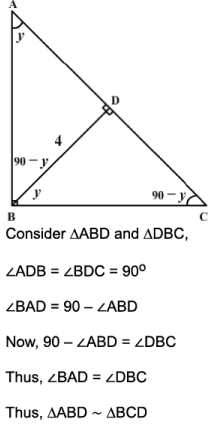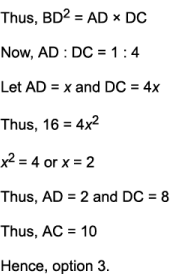QUESTION: 18

A cow is tethered, using a rope of 20 ft, to a corner of a rectangular house which measures 16 ft by 10 ft, situated in the middle of a very big grass land. What is the maximum area that can be grazed by the cow?

Solution:

Refer the diagram,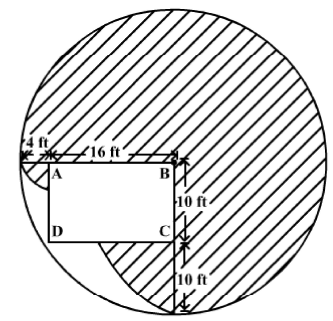The shaded region represents the maximum area that can be grazed by the cow.
Let the cow be tethered to the top right corner (point B) of the rectangular house ABCD.
From the figure, it can be seen that:

i. The cow can graze 3/4th of the area of the circle of radius 20 ft.

ii. Moving to the left, along the length of the house the rope can go up to 16 ft along the boundary of the house. The remaining 4 ft of the rope would enable the cow to graze a small quarter circle of radius 4 ft. iii. Moving down, along the breath of the house, the rope can go up to 10 ft along the boundary of the house. The remaining 10 ft of the rope would enable the cow to graze another quarter circle of radius 10 ft.
The maximum total area that the cow can graze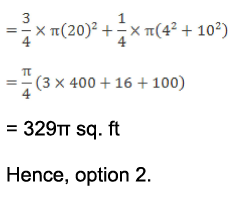QUESTION: 19

Group Question

Answer the following question based on the information given below.

The questions are based on the following pie charts: Following pie charts give the details of the number of students in the age group of 25 to 27 years, pursuing different specializations in September 2007 and March 2008 at an MBA college. No student joined or left the college or made a shift to another specialization during this period.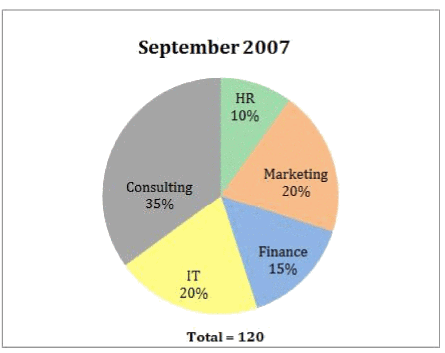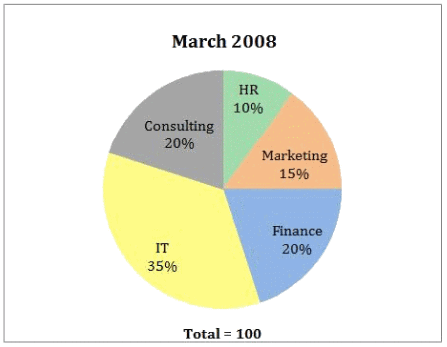Q. The minimum number of students who crossed the age of 27 between September 2007 and March 2008 is:

Solution: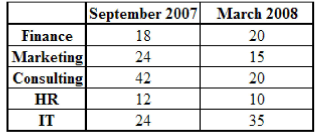The number of students in different specialisations in the age group of 25 years to 27 years in September 2007 and March 2008 are as shown in the table.
The number of students in each area of specialisation in March 2008 = Number of students in that area in September 2007 + Number of students in that area, who attain the age of 25 by March 2008 - Number of students in that area, who cross the age of 27 by March 2008 From the table, at least 24 - 15 = 9 students in marketing, 42 - 20 = 22 students in Consulting, and 12 - 10 = 2 students in HR crossed the age of 27 years between September 2007 and March 2008.
Hence, option 2.

QUESTION: 20

The questions are based on the following pie charts: Following pie charts give the details of the number of students in the age group of 25 to 27 years, pursuing different specializations in September 2007 and March 2008 at an MBA college. No student joined or left the college or made a shift to another specialization during this period.Q. Which is the most popular specialization in the age group 24 years 6 months to 25 years in September 2007?

Solution:

To find the exact number of students in the age group 24 years 6 months to 25 years in September 2007, we need to know the number of students who crossed the age of 27 years in that period.
Hence, option E.

QUESTION: 21

The questions are based on the following pie charts: Following pie charts give the details of the number of students in the age group of 25 to 27 years, pursuing different specializations in September 2007 and March 2008 at an MBA college. No student joined or left the college or made a shift to another specialization during this period.Q. If the number of students aged 26 years 6 months to 27 years in September 2007, was the minimum possible, then which of the following statements is definitely true?

Solution:

If the number of students in the age group 26 years 6 months to 27 years in September 2007 was the minimum possible then a minimum number of students would have crossed the age of 27 years (which is 33 from the first question).
It can be seen that only option 3 is correct.
Hence, option 3.

QUESTION: 22

The questions are based on the following pie charts: Following pie charts give the details of the number of students in the age group of 25 to 27 years, pursuing different specializations in September 2007 and March 2008 at an MBA college. No student joined or left the college or made a shift to another specialization during this period.Q. If the number of students who attained the age of 25 years between September 2007 and March 2008 and specialising in finance was the minimum possible, it was

Solution:

As the number of students who attained the age of 25 years was the minimum possible, it must be 2 (20 - 18).
Hence, option 2.

QUESTION: 23

The questions are based on the following pie charts: Following pie charts give the details of the number of students in the age group of 25 to 27 years, pursuing different specializations in September 2007 and March 2008 at an MBA college. No student joined or left the college or made a shift to another specialization during this period.Q. If the number of students who crossed the age of 27 years between September 2007 and March 2008 and specialising in Marketing was more than in any other specialisations, what is the minimum number of students in that specialisation who crossed the age of 25 years between September 2007 and March 2008?

Solution:

The number of students in each area of specialisation in March 2008 = Number of students in that area in September 2007 + Number of students in that area, who attain the age of 25 by March 2008 - Number of students in that area, who cross the age of 27 by March 2008 Consider Consulting, 20 = 42 + x - y. y - x = 22 The minimum value of y here is 22. Considering Marketing, 15 = 24 + x - y. y - x = 9

y has to be greater than 22.
Minimum value of y = 23 Minimum value of x= 14 Hence, option 2.

QUESTION: 24

Group Question

Answer the following question based on the information given below.

The table given below shows the age-wise population distribution of India in 2010.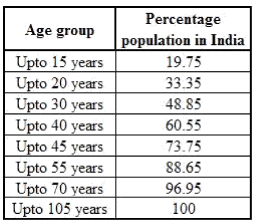Q. In 2011, the population of only the age group 41 to 50 years increases, while population of other groups remains constant. If the population of the age group 41 to 50 years, in 2011, increases by 15% over that in 2010, then what is the ratio of the minimum percentage increase and maximum percentage increase in India’s total population?

Solution:

Let India’s population in 2010 be 100.
Hence, population of age group 41 to 45 years = 73.75 - 60.55 = 13.2
Hence, population of age group 41 to 50 was at least 13.2.
Now, population of age group 46 to 55 years is 88.65 - 73.75 = 14.9
Hence, maximum and minimum number of people in age group 46 to 50 is 14.9 and 0 respectively. Hence, maximum and minimum population of age group 41 to 50 years is 28.1 and 13.2 respectively.
Now, as population of only age group 41 to 50 increases, hence percentage increase in India’s total population is directly proportional to population of this group.
Hence, the required ratio = 13.2 : 28.1 « 0.469 : 1 Hence, option 2.

QUESTION: 25

The table given below shows the age-wise population distribution of India in 2010.Q. Which of the following age-groups account for maximum population?

Solution:

Let India's population, in 2010, was 100.
Hence,
Population of age group 31 to 40 years = 60.55 - 48.85 = 11.7
Population of age group more than 55 years = 100 - 88.65 = 11.35
Population of age group 16 to 20 years = 33.35 - 19.75 = 13.6
Population of age group upto 15 years = 19.75 Population of age group 41 to 45 years = 73.75 - 60.55 = 13.2

Hence, age group below 15 accounts for the highest population among the given age groups. Hence, option 4.

QUESTION: 26

The table given below shows the age-wise population distribution of India in 2010.Q. What is the difference between the population in the age- group of 15 - 20 years and 40 - 45 years assuming that 25 million people are in the age-group of 56 and above?

Solution:

From the table, Population in the age-group of 56 and above accounts for 11.35% of total population.
Let ‘x' be the total population (in million)of the state.
Then, 11.35% of x = 25, x = 220.26. Population in the age-group of 15 - 20 years accounts for 13.6% (i.e. 33.35% - 19.75%) of total population.
Population in the age-group of 40 - 45 years accounts for 13.2 % (i.e. 73.75% - 60.55%) of total population.

Required difference (in million) = (13.6% of x) - (13.2% of x) = 0.4% of 220.26

= 0.88 Or = 8.8 lakhs Hence, option 4.

QUESTION: 27

The table given below shows the age-wise population distribution of India in 2010.Q. Which of the following represents the ratio of populations of  the age-group of upto 30 years and age-group of above 55 years?

Solution:

From the table, Population of age-group upto 30 years accounts for 48.85% of total population.
Population of age-group above 55 years accounts for 11.35% of total population Required ratio = 48.85 : 11.35 ⇒ 1 : 0.232
Hence, option 4.

QUESTION: 28

Each question is followed by two statements, A and B. Choose the most appropriate option given below.

What is the value of (x + y) if ( x - y )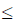5?

A. 2x + 3= 17

B. 2(x + 2) - 3(y - 2) = 31

Solution:

From statement A alone: When x = 4 and y = 0 , 2x + 3y= 24 + 30 = 16 + 1 = 17 and x + y = 4 + 0 = 4
When x = 3 and y = 2, 2x + 3y = 23 + 32 = 8 + 9 = 17 and x + y = 3 + 2 = 5
In both the possibilities, x + y is not same.
Statement A alone is not sufficient as it does give us an unique answer.

From statement B alone: We have only one possibility.
When x = 3 and y = 2, 2(x + 2) - 3(y- 2)= 2(3+2) - 3(2 - 2) = 25 - 30 = 32 - 1 = 31
x + y = 3 + 2 = 5. Statement B alone is sufficient to answer this question. Hence, option 2.

QUESTION: 29

Amar and Baron are given a six-shot pistol which has only one bullet in its cylinder. Both of them fire a shot one after the other.A shot can be a blank shot or a lethal shot. The one, who fires a lethal shot, is the winner. After each shot the cylinder of the pistol is shuffled so that the other person has a fair chance of firing a lethal shot. It is also known that Amar had the opportunity to fire the first shot. What is the probability that Baron wins the game?

Solution:

Let probability that Amar wins the match be a and probability that Baron wins the match is b. a + b = 1 ... (i) Baron will get a chance to shoot only if Amar has a blank shot.
Now, probability of Amar firing a blank shot is 5/6.
After Amar’s blank shot, the cylinder of the pistol is shuffled.

Hence, probability that Baron shoots a lethal shot is same as that of Amar. b = (5/6) x a Substituting this value in equation (i), we get, a + (5/6)a = 1 a = 6/11 Hence, b = 5/11 Hence, option 4.Use Code STAYHOME200 and get INR 200 additional OFF Use Coupon Code

How to Prepare for CAT

Read our guide to prepare for CAT which is created by Toppers & the best Teachers

Track your progress, build streaks, highlight & save important lessons and more!

Similar ContentRelated tests